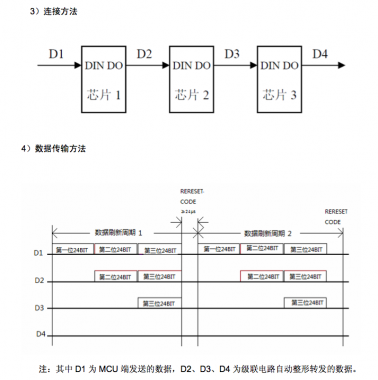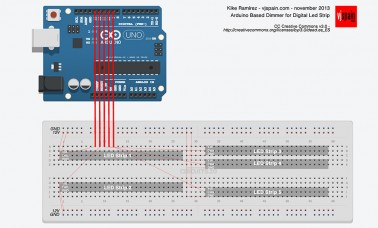# Project: Arduino Based Dimmer for Digital LED Strips

Arduino Based Dimmer for Digital LED Strip (1903 IC controlled)

(Spanish version HERE)

Target

In this tutorial we will use an Arduino, connected via USB to a laptop to control 5 segments of digital LED strip. In order to manage each segment flashing, we will use a Processing sketch which will be running simultaneously to analyze sounds, detect beats and send string commands to Arduino via USB serial port.

Materials and Software:

–       5 segments of 1 meter each of LED Strip (1903 IC or compatible controlled). Example.
–       1 Arduino UNO. Reference.
–       1 12V Voltage supply. Example.
–       1 USB wire. Example.
–       1 computer with Processing and Arduino IDE installed.
–       Processing libraries MINIM and Serial.
–       Arduino library FastSPI_LED.

Digital LED Strips

Digital LED strips are similar to RGB LED strips, but digital allows to control each LED (or groups of N LEDs) indenpendently. The advantage of it is an enormous saving of wiring (and money): in this example we are controlling 75 groups of LED with only five Arduino outputs and five wiring.

In the other hand, digital LED strips have a higher delay to update every LED, since the information passes linearly from first element to each another until the last one. In those installations where high lengths of digital LEDs strips must be covered, the delay may be noticeable. In this cases we recommend to divide the total lenght in shorter segments to optimize the relationship delay/wiring.

Digital LED Strips are controlled by a microcontroller (1903 IC in this case) which decodes Data Signal pulses and outputs the PWM signal that controls R, G and B pins of every LED.Internal conexion of LED Strip scheme. Each element gets the whole set of commands, takes the one assigned to him, and pass forward the rest of information. In longth strips this may cause high delays.

DATASHEET (Japanese) of UCS1903 microcontroller: UCS1903. (Some Google Translator use may help to understand something).

Fast_SPI2 Arduino Library

This library, published under a MIT License, offers a easy way to control digital LED strips controlled by different microchips. You can check the list of supported chipset is available here: https://code.google.com/p/fastspi/wiki/ChipsetOverview. In our case, the chipset compatible to UCS1903 is WS2811.

In order to select the correct microcontroller you should create a LEDS objetct from Fast SPI LED Library in the following way:

`LEDS.addLeds<MICROCONTROLLER, PIN>(COLOR_DATA, NUM_LEDS);`

Example:

`LEDS.addLeds<WS2811, 13>(leds, 15);`

It’s functionality is simple and it offers some Basic examples to start playing instantaneously with LEDs.

Its working cycle is:

`Update LED color values -> Turn ON LEDs -> delay N microseconds -> Turn OFF LEDs`

An example:

`for(int i = 0; i < 3; i++) {`
`for(int iLed = 0; iLed < NUM_LEDS; iLed++) {`
`memset(leds, 0,  NUM_LEDS * sizeof(struct CRGB));`
` `
`switch(i) {`
`// You can access the rgb values by field r, g, b`
`case 0: leds[iLed].r = 128; break;`
` `
`// or by indexing into the led (r==0, g==1, b==2)`
`case 1: leds[iLed][i] = 128; break;`
` `
`// or by setting the rgb values for the pixel all at once`
`case 2: leds[iLed] = CRGB(0, 0, 128); break;`
`}`
` `
`// and now, show your led array!`
`LEDS.show();`
`delay(10);`
`}`

Our system:

The connection is really simple. The power supply will be connected to LED strips which requires higher currents. Arduino can be voltage supplied via USB wire.

In Arduino sketch (attached below) we define pins from 9 to 13 as output and will be directly connected to data pins of every LED strip.In this schema you can notice how the output pins declared in Arduino Sketch (from 9 to 13) are directly connected to each segment’s data pin. (Click to enlarge)

Arduino Sketch

You can find a zip file here: vjspain_ArduinoLedDimmer.ino.

`/*`
`Kike Ramirez - vjspain.com, november, 2013`
```Arduino based Dimmer controlling 5 segments of digital LED Strips with microcontroller WS2811.
Each segment contains 15 (NUM_LEDS) groups of 3 independents LEDs.```
`CC Creative Commons v3.0 - http://creativecommons.org/licenses/by/3.0/deed.es_ES`
`*/`
`#include "FastSPI_LED2.h"`
```// Number of independents LEDs in each strip segment
#define NUM_LEDS 15```
```// Output PIN (Data LED strip connection) definition
#define DATA_PIN1 13
#define DATA_PIN2 12
#define DATA_PIN3 11
#define DATA_PIN4 10
#define DATA_PIN5 9```
```// This is an array of leds. One item for each led in your strip. 5 segments in this case.
CRGB leds1[NUM_LEDS];
CRGB leds2[NUM_LEDS];
CRGB leds3[NUM_LEDS];
CRGB leds4[NUM_LEDS];
CRGB leds5[NUM_LEDS];```
```// Data reception via serial counter.
int incomingByte = 0;```
```// String buffer
```byte contador=0;
int valor=0;```
```// Flags
boolean sb1, sb2, sb3, sb4;```
`void setup() {`
```// Opens serial oprt and set 9600bps
Serial.begin(9600);

// Initialize each segment
`}`
```void loop() {

// Initialize flags
sb1 = false;
sb2 = false;
sb3 = false;
sb4 = false;```
` if (Serial.available() >= 4) // this was == 4, more than one command may be waiting`
```// Memory allocation
```{
for (int i=0; i < 4; i++)
{
}

if (strcmp(cadena, " B1") == 0) // If B1 is received, update in red.
{

sb1 = true;
destello(255,0,0);

}

else if (strcmp(cadena, " B2") == 0) // If B2 is received, update in green
{

sb2 = true;
destello(0,255,0);

}

else if (strcmp(cadena, " B3") == 0) // If B3 is received, update in blue
{

sb3 = true;
destello(0,0,255);

}

else if (strcmp(cadena, " B4") == 0) // If B4 is received, update in white
{

sb4 = true;
destello(255,255,255);

}```
``` }

// Once updated, flash it!
pulsoLeds();
}```
` `
```// Funcion to turn all LEDs off
void apagarLeds() {

for (int i = 0; i < NUM_LEDS; i++) {

leds1[i].r = 0;
leds1[i].g = 0;
leds1[i].b = 0;

}```
```for (int i = 0; i < NUM_LEDS; i++) {

leds2[i].r = 0;
leds2[i].g = 0;
leds2[i].b = 0;

}

for (int i = 0; i < NUM_LEDS; i++) {

leds3[i].r = 0;
leds3[i].g = 0;
leds3[i].b = 0;

}```
```for (int i = 0; i < NUM_LEDS; i++) {

leds4[i].r = 0;
leds4[i].g = 0;
leds4[i].b = 0;

}

for (int i = 0; i < NUM_LEDS; i++) {

leds5[i].r = 0;
leds5[i].g = 0;
leds5[i].b = 0;

}
}```
```// Function to flash all LEDs
void pulsoLeds() {

FastLED.show();
delay(10);
apagarLeds();
FastLED.show();

}```
```// Function to update LEDs with a colour
void destello(int r, int g, int b) {

for (int i = 0; i < NUM_LEDS; i++) {

leds1[i].r = r;
leds1[i].g = g;
leds1[i].b = b;

}```
```for (int i = 0; i < NUM_LEDS; i++) {

leds2[i].r = r;
leds2[i].g = g;
leds2[i].b = b;

}```
```for (int i = 0; i < NUM_LEDS; i++) {

leds3[i].r = r;
leds3[i].g = g;
leds3[i].b = b;

}```
```for (int i = 0; i < NUM_LEDS; i++) {

leds4[i].r = r;
leds4[i].g = g;
leds4[i].b = b;

}```
```for (int i = 0; i < NUM_LEDS; i++) {

leds5[i].r = r;
leds5[i].g = g;
leds5[i].b = b;

}

}```

The sketch is quite simple: it just «hears» continuously through USB Serial and receives every string message. Depending on the command received (possibilities are B1, B2, B3 and B4), it flashes in red, green, blue or white.

It implements the functions:

• apagarLeds(), which turns off all the elements.
• destello() function receive a color as an in put and update the memory values for every LED in its corresponding color.
• pulsoLeds() just turns ON and OFF LEDs the enough time to perceive it, it make a flash

Processing Sketch

`/*`
`Kike Ramirez - vjspain.com, november, 2013`
`Sound Analysis Skech. Beat Detector used to send serial data to an Arduino connected via USB to control digital LED Strips.`
`CC Creative Commons v3.0 - http://creativecommons.org/licenses/by/3.0/deed.es_ES`
`*/`
```import ddf.minim.*;
import ddf.minim.analysis.*;
import processing.serial.*;```
```// Declarations
Minim minim;
AudioInput in;
BeatDetect beat;
Serial myPort;```
```void setup()
{
size(512, 200, P3D);

// Serial port opening at 9600bps
myPort = new Serial(this, Serial.list(), 9600);

// Initialize Minim object
minim = new Minim(this);

// use the getLineIn method of the Minim object to get an AudioInput
in = minim.getLineIn();

// Initialize beat detector to 1024 samples and 44100 samples per second
beat = new BeatDetect(1024,44100);

// uncomment this line to *hear* what is being monitored
// in.enableMonitoring();
}```
```void draw()
{
background(0);
stroke(255);

// draw the waveforms so we can see what we are monitoring
for(int i = 0; i < in.bufferSize() - 1; i++)
{
line( i, 50 + in.left.get(i)*50, i+1, 50 + in.left.get(i+1)*50 );
line( i, 150 + in.right.get(i)*50, i+1, 150 + in.right.get(i+1)*50 );
}

// Draw beat detection as a rectangle
beat.detect(in.left.toArray());

// If "Hat" is detected sent B1
if (beat.isHat()) {

fill(255,0,0);
rect(0,0,20,20);
myPort.write(" B1");

}```
```// If "Kick" is detected sent B2
if (beat.isKick()) {

fill(0,255,0);
rect(0,30,20,20);
myPort.write(" B2");

}```
```// If "Onset" is detected sent B3
if (beat.isOnset()) {

fill(0,0,255);
rect(0,60,20,20);
myPort.write(" B3");

}```
```// If "Snare" is detected sent B4
if (beat.isSnare()) {

fill(255);
rect(0,90,20,20);
myPort.write(" B4");```
```}

}
```

The Processing sketch is also really basic. Let’s ennumerate what it does:

• It monitorizes the internal microphone of the computer continuously.
• Draws the «amplitud vs time» representation of the sound captured.
• Use BeatDetect class from Minim library which detects different type of beats depending on the power/frequency of the sound. In order to know more about this type of beats, check here.
• For each beat event, it draws a rectangle in the sketch canvas in the appropiate color.
• Sends the proper command to Arduino via Serial USB port.

Why this configuration?

Why not using Arduino in stand-alone configuration with a microphone connected and doing all the analysis internally? Why do we need a computer with a processing sketch running at the same time?

We are aware that this may not be the optimal configuration for controlling some digital LED Strips, but it is on half way to where we want to reach.

Why should we create a LED controller software with all the possible options and effect, with a complex interface, etc. when we can find multiple softwares like madMapper and hundreds of DMX software which already offers this option?

We are just creating a Digital LED Strip Dimmer. And the final goal of this project is creating a DMX interface, so our dimmer can be connected to an ENTTEC and me managed directly using the complete functionality of MadMapper or any VDMX Controller, just as a normal dimmer with an RGB LED Strip.

That is the reason why we choosed to start creating a Dimmer controlled via Serial by a Processing Sketch. It is just a first step.

We will keep you informed about the evolution of the project. Comments and suggestions are, as always, welcomed!!!